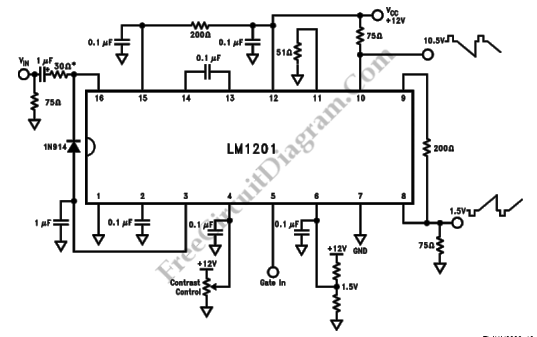# LM1201 Video Amplifier with Balanced (Bi-Phase) OutputThis is a schematic diagram of video amplifier circuit with bi-phase output.  Bi-phase output provides both positive-going and negative-going signal, and can be used as balanced signaling. The main component of this circuit is LM1201.  In this circuit, the inverted video is obtained at pin 10 because of the 75Ω termination to the power supply voltage. The voltage divider on pin 6 is used to set the black level on inverted video (pin 8) to 1.5V. Here is the schematic diagram of the circuit:This is a Video Amplifier circuit with Bi-Phase Output. The maincomponent of this circuit is LM1201. The inverted video can be

obtained at pin 10 because of the 75Ω termination to the power

supply voltage. The voltage divider on pin 6 is used to set the

Black level on inverted video(pin 8) to 1.5V. Here is the circuit:This is a Video Amplifier circuit with Bi-Phase Output. The main

component of this circuit is LM1201. The inverted video can be

obtained at pin 10 because of the 75Ω termination to the power

supply voltage. The voltage divider on pin 6 is used to set the

Black level on inverted video(pin 8) to 1.5V. Here is the circuit:This is a Video Amplifier circuit with Bi-Phase Output. The main

component of this circuit is LM1201. The inverted video can be

obtained at pin 10 because of the 75Ω termination to the power

supply voltage. The voltage divider on pin 6 is used to set the

Black level on inverted video(pin 8) to 1.5V. Here is the circuit:This is a Video Amplifier circuit with Bi-Phase Output. The main

component of this circuit is LM1201. The inverted video can be

obtained at pin 10 because of the 75Ω termination to the power

supply voltage. The voltage divider on pin 6 is used to set the

Black level on inverted video(pin 8) to 1.5V. Here is the circuit: